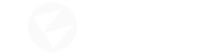# World Displacement and its effect on the texture

Hello. When I use world displacement, I want the scene texture to be affected as well. But when I use scene texture, I have to choose the screen coordinate for its uv. This time, the texture is not affected by displacement. How do I achieve this? Since my English is not good, I prepared a video to explain the problem better. Thanks in advance.

in DX9 I was doing like this;

``````//VERTEX SHADER
//before displacement
float4 pM= mul(In.Pos,matWorldViewProj);
Out.Tex1=pM;
tex2Dlod...etc..

float3 refract_uv;
refract_uv.x = 0.5 * (i.Tex1.w + i.Tex1.x);
refract_uv.y = 0.5 * (i.Tex1.w - i.Tex1.y);
refract_uv.z = i.Tex1.w * 1;
float4 waterRefract=tex2D(refractSampler,refract_uv.xy/refract_uv.z);
``````

In the Material editor, however, some things seem to be limited. As far as I know, there is no way I can do such a thing. What do you guys recommend?

Where do you sample this Screen Coordinate? Is it for vertex related math (eg. Position Offset or Tess Mul)? because the World Displacement is evaluated in Domain Shader (see docs).

Screen Texture uses `SvPosition / ScreenSize` to calculate the texcoord for a shaded Pixel.

Yes, it is vertex related. (let’s say position offset) The important point here is to be able to access ` mul(pos,matWorldViewProj)` before vertex manipulation. In this way, the relevant UV can be affected by vertex manipulation. Let me exemplify from directx9:

``````VS_OUTPUT VS_p0(VS_INPUT In)
{

VS_OUTPUT Out;

//for refraction uv --Get Out.Pos BEFORE vertex manipulation--
Out.Tex1= mul(In.Pos,matWorldViewProj);

//vertex manipulation--> tex2Dlod(DX9) a.k.a SampleLevel(DX11)
In.Pos.y+=tex2Dlod(HeightMap,float4(In.Tex0.xy,0,0))*50;

//Set Out.Pos
Out.Pos=mul(In.Pos,matWorldViewProj);
return Out;
}
float4 ComputeSeaPS(VS_OUTPUT i) : COLOR
{

float3 refraction_uv;
//i.Tex1== mul(In.Pos,matWorldViewProj))
refraction_uv.x = 0.5 * (i.Tex1.w + i.Tex1.x);
refraction_uv.y = 0.5 * (i.Tex1.w - i.Tex1.y);
refraction_uv.z = i.Tex1.w * 1;

float4 refraction=tex2D(RefractionMap,(refraction_uv.xy/refraction_uv.z));

return refraction;

}
``````

Edit: I am unfamiliar with new technology. If it’s not possible or very difficult to do this in dx11, it’s fine. I wouldn’t want you to waste your time on just one small feature.